Courses

# Design Principles For Thick Cylinders (Part - 1) Mechanical Engineering Notes | EduRev

## Mechanical Engineering : Design Principles For Thick Cylinders (Part - 1) Mechanical Engineering Notes | EduRev

The document Design Principles For Thick Cylinders (Part - 1) Mechanical Engineering Notes | EduRev is a part of the Mechanical Engineering Course Machine Design.
All you need of Mechanical Engineering at this link: Mechanical Engineering

Application of theories of failure for thick walled pressure vessels.

Having discussed the stresses in thick walled cylinders it is important to consider their failure criterion. The five failure theories will be considered in this regard and the variation of wall thickness to internal radius ratio t/ri or radius ratio ro/ri with p/σyp for different failure theories would be discussed. A number of cases such as po =0, pi =0 or both non-zero po and pi are possible but here only the cylinders with closed ends and subjected to an internal pressure only will be considered, for an example.

9.3.1.1 Maximum Principal Stress

theory According to this theory failure occurs when maximum principal stress exceeds the stress at the tensile yield point. The failure envelope according to this failure mode is shown in figure-9.3.1.1.1 and the failure criteria are given by σ1 = σ= ± σyp. If po =0 the maximum values of circumferential and radial stresses are given by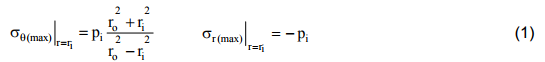Here both σθ and σr are the principal stresses and σθ is larger. Thus the condition for failure is based on σθ and we have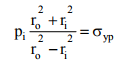where σyp is the yield stress.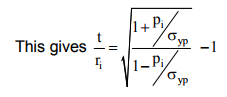(2)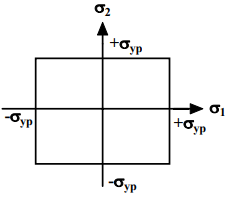9.3.1.1.1F- Failure envelope according to Maximum Principal Stress Theory.

9.3.1.2 Maximum Shear Stress

theory According to this theory failure occurs when maximum shear stress exceeds the maximum shear stress at the tensile yield point. The failure envelope according to this criterion is shown in figure- 9.3.1.2.1 and the maximum shear stress is given by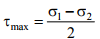where the principal stresses σ1 and σ2 are given by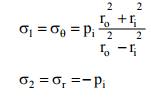Here σ1 is tensile and σ2 is compressive in nature. τmax may therefore be given by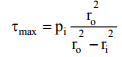(3) and since the failure criterion is τmax = σyp / 2 we may write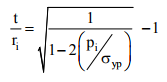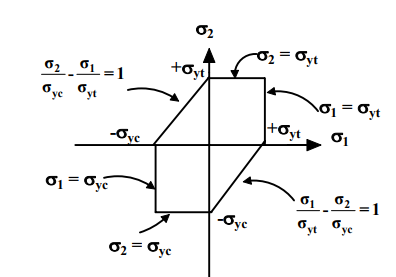9.3.1.2.1F- Failure envelope according to Maximum Shear Stress theory.

9.3.1.3 Maximum Principal Strain theory
According to this theory failure occurs when the maximum principal strain exceeds the strain at the tensile yield point.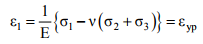and this gives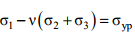where εyp and σyp are the yield strain and stress respectively. Following this the failure envelope is as shown in figure-9.3.1.3.1. Here the three principle stresses can be given as follows according to the standard 3D solutions: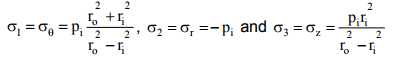(5)

The failure criterion may now be written as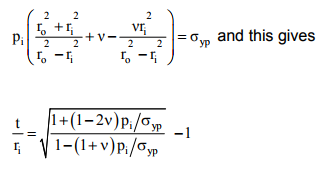(6)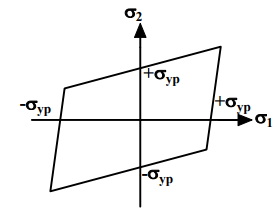9.3.1.3.1F- Failure envelope according to Maximum Principal Strain theory

9.3.1.4 Maximum Distortion Energy

Theory According to this theory if the maximum distortion energy exceeds the distortion energy at the tensile yield point failure occurs. The failure envelope is shown in figure-9.3.1.4.1 and the distortion energy Ed is given by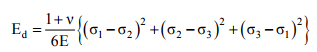Since at the uniaxial tensile yield point σ= σ= 0 and σ1 = σyp
Ed at the tensile yield point

We consider σ1 = σθ , σ2 = σr and σ= σand therefore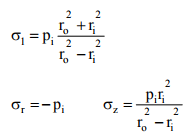(7)

The failure criterion therefore reduces to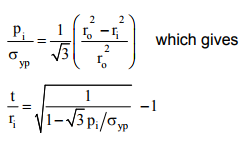(8)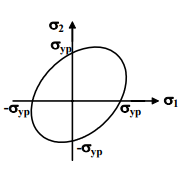9.3.1.4.1F- Failure envelope according to Maximum Distortion Energy Theory

Plots of pi/σyp and t/ri for different failure criteria are shown in figure9.3.1.4.2.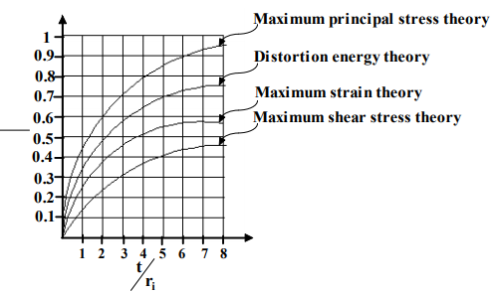9.3.1.4.2F- Comparison of variation of against t/ri for different failure criterion.

The criteria developed and the plots apply to thick walled cylinders with internal pressure only but similar criteria for cylinders with external

pressure only or in case where both internal and external pressures exist may be developed. However, on the basis of these results we note that the rate of increase in piyp is small at large values of t/ri for all the failure modes considered. This means that at higher values of pi small increase in pressure requires large increase in wall thickness. But since the stresses near the outer radius are small, material at the outer radius for very thick wall cylinders are ineffectively used. It is therefore necessary to select materials so that pi/σyp is reasonably small. When this is not possible prestressed cylinders may be used.

All the above theories of failure are based on the prediction of the beginning of inelastic deformation and these are strictly applicable for ductile materials under static loading. Maximum principal stress theory is widely used for brittle materials which normally fail by brittle fracture.

In some applications of thick cylinders such as, gun barrels no inelastic deformation can be permitted for proper functioning and there design based on maximum shear stress theory or maximum distortion energy theory are acceptable. For some pressure vessels a satisfactory function is maintained until inelastic deformation that starts from the inner radius and spreads completely through the wall of the cylinder. Under such circumstances none of the failure theories would work satisfactorily and the procedure discussed in section lesson 9.2 is to be used.

9.3.1.5 Failure criteria of pre-stressed thick cylinders

Failure criteria based on the three methods of pre-stressing would now be discussed. The radial and circumferential stresses developed during shrinking a hollow cylinder over the main cylinder are shown in figure9.3.1.5.1.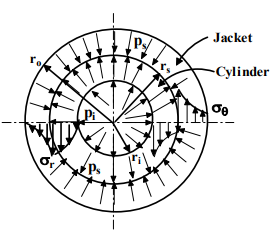9.3.1.5.1F- Distribution of radial and circumferential stresses in a composite thick walled cylinder subjected to an internal pressure.

Following the analysis in section 9.2 the maximum initial (residual) circumferential stress at the inner radius of the cylinder due to the contact pressure ps is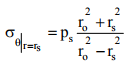and the maximum initial (residual) circumferential stress at the inner radius of the jacket due to contact pressure ps is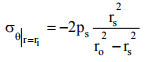Superposing the circumferential stresses due to pi (considering the composite cylinder as one) the total circumferential stresses at the inner radius of the cylinder and inner radius of the jacket are respectively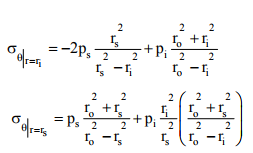These maximum stresses should not exceed the yield stress and therefore we may write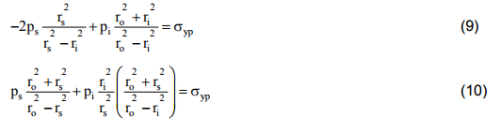It was shown in section-9.2 that the contact pressure ps is given by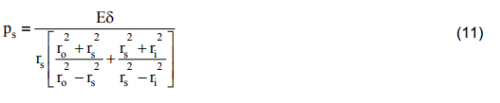From (9), (10) and (11) it is possible to eliminate ps and express t/rin terms of piyp and this is shown graphically in figure-9.3.1.5.2.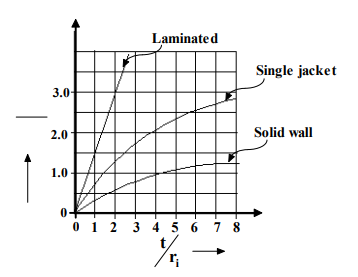9.3.1.5.2F- Plot of piyp vs t/ri for laminated multilayered, single jacket and solid wall cylinders.

Offer running on EduRev: Apply code STAYHOME200 to get INR 200 off on our premium plan EduRev Infinity!

## Machine Design

57 videos|71 docs|102 tests

,

,

,

,

,

,

,

,

,

,

,

,

,

,

,

,

,

,

,

,

,

;